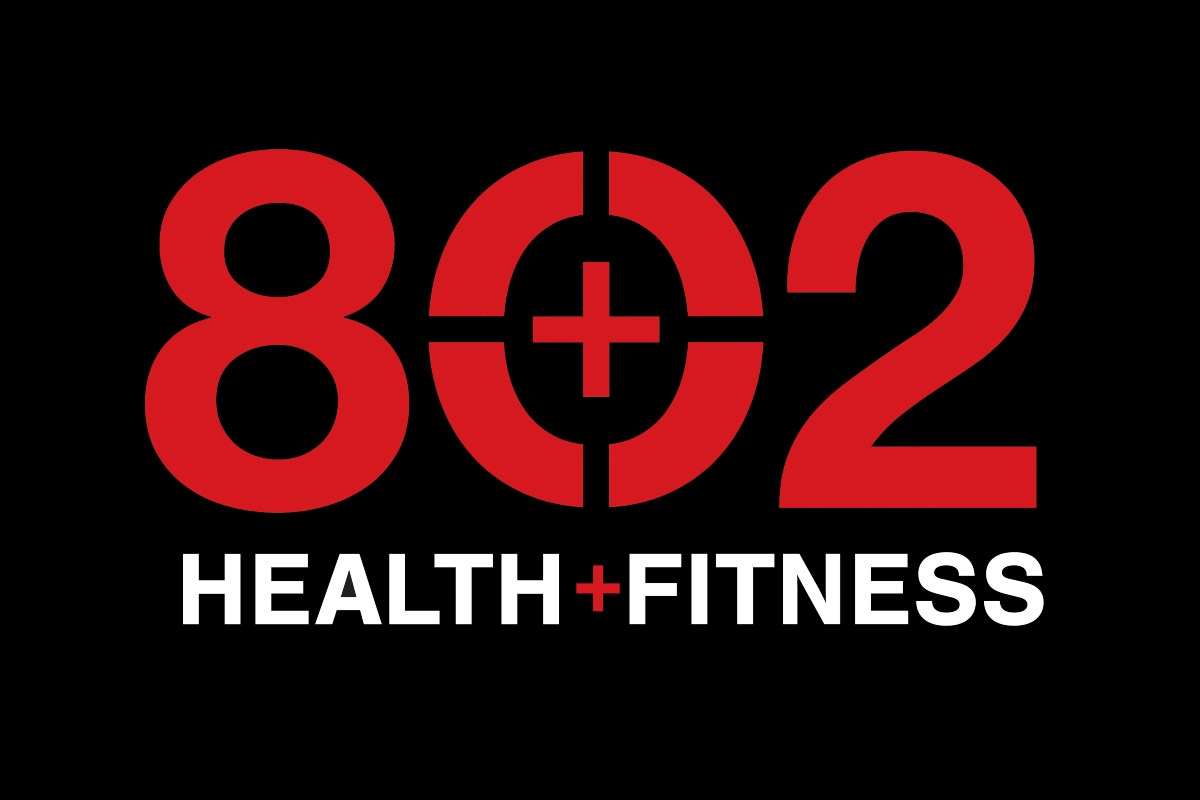# Wednesday 5/26/21

By
September 27, 2022## The WOD

This WOD is a build. Every 3 rounds, another movement will be added to the list. The score-able portion comes from the final 3 rounds only, where you will do as many burpees as possible with the remainder of 3 minutes.Round 1, 2, 3 (3:00 loop)

• 1 Hang Clean + 1 Push Jerk X3
• + Double Unders (Rx=30, Per=20, Fit=0:20 of attempts)
• + Run (Rx=80m, Per=60m, Fit=40m)
• +V-Ups(Rx=10, Per=8, Fit=6 V2Shin)

Round 4, 5, 6 (3:00 loop)

• 1 Hang Clean + 1 Push Jerk X3
• + Double Unders (Rx=30, Per=20, Fit=0:20 of attempts)
• + Run (Rx=80m, Per=60m, Fit=40m)
• +V-Ups(Rx=10, Per=8, Fit=6 V2Shin)
• + Tuck-Up (Rx=10, Per=8, Fit=6 )

Round 7, 8, 9 (3:00 loop)

• All Above
• + Air Squats (Rx=10, Per=8, Fit=6 )

Round 10, 11, 12 (3:00 loop)

• All Above
• + As Many Burpees as Possible in remainder of 3:00 loop

### The Finisher

(optional)Shrugs (w/ DB)